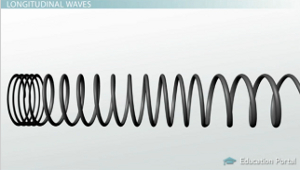# What are longitudinal and transverse wave? and is Sound a longitudinal wave?

A wave in which the vibration of the particles is along the direction of propagation of wave in a to and fro fashion is called longitudinal wave.

Example: Compression and rarefaction waves in a spring, sound waves.

A wave in which the vibration of the particles (in case of mechanical wave) is perpendicular to the direction of propagation of wave is called a transverse wave (In case of electromagnetic wave the oscillation of electric and magnetic field are perpendicular to the direction of propagation of the wave).

Example: Water waves, electromagnetic waves.

Yes, sound is a longitudinal wave.

• 2

longitudinal waves:when the particles of the medium vibrate parallel to the direction of wave motion,then it is a longitudinal wave.

transverse waves:when the particles of the medium vibrate perpendicular to the direction of wave motion,then it is a transverse wave.

yes, sound wave is longitudinal and light waves are transversal.• 2

transverse wave : when the particles of the medium vibrate perpendicular to the direction of wave motion,then it is a transverse wave. u can imagine about the ripples formed when u throw a stone in water .here the waves go in this way as given in the following diagram .... always remember that they are continuous ....andlongitudinal waves:when the particles of the medium vibrate parallel to the direction of wave motion,then it is a longitudinal wave. here u can imagine the compression of a spring,,the waves are such in longitudal wave .......yes sound is longitudal......

the difference between both is as follows............• 0
What are you looking for?